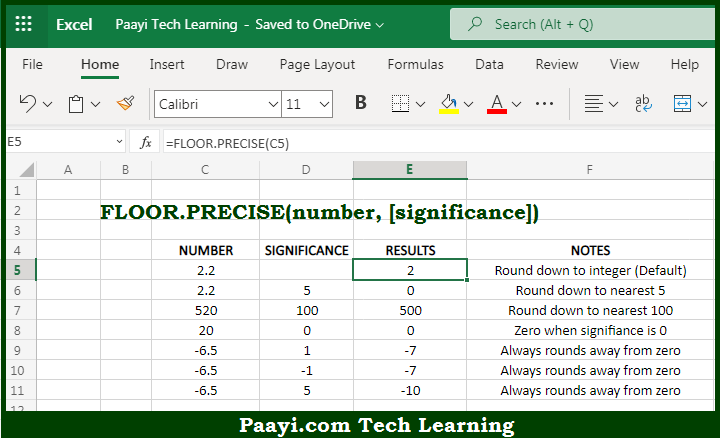# Learn How to Use Microsoft Excel FLOOR.PRECISE Function

Written by | 0 Comments | 558 Views

In this article, you will learn how to use the Microsoft Excel FLOOR.PRECISE function and its prime function in Microsoft Excel. You will also get to know the Microsoft Excel FLOOR.PRECISE function return value and syntax with the help of some examples.

Microsoft Excel FLOOR.PRECISE Function

The main function of Microsoft Excel FLOOR.PRECISE function is to round down the number to the nearest multiple. So, with the help of FLOOR.PRECISE function, you can able to round up the given number, to the nearest specified multiple. It should be noted that, the FLOOR.PRECISE is different as compared with FLOOR function, as FLOOR.PRECISE defaults to a multiple of 1 and offer complete support for rounding negative numbers. So, with the help of Microsoft Excel FLOOR.PRECISE function you can easily round down the number to the nearest multiple.

Return Value of FLOOR.PRECISE Function

The return value will be the round-down number.

Syntax of FLOOR.PRECISE Function

=FLOOR.PRECISE(number, [significance])

Where the arguments:

• number: This is the number of which you want to round down.
• significance: This is multiple to use while rounding down the number, the default is 1 (optional).

## How to Use Microsoft Excel FLOOR.PRECISE Function?So we know that, Microsoft Excel FLOOR.PRECISE function you can able to round up the given number, to the nearest specified multiple. Positive numbers with decimal values are rounded down to the nearest integer, for example,  5.7 is rounded down to 5, while negative numbers with decimal values are rounded away from zero for example, -6.8 is rounded to -7.

It should be noted that, the FLOOR.PRECISE is different as compared with FLOOR function, as FLOOR.PRECISE defaults to a multiple of 1 and offer complete support for rounding negative numbers. So, with the help of Microsoft Excel FLOOR.PRECISE function you can easily round down the number to the nearest multiple.